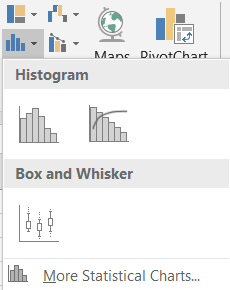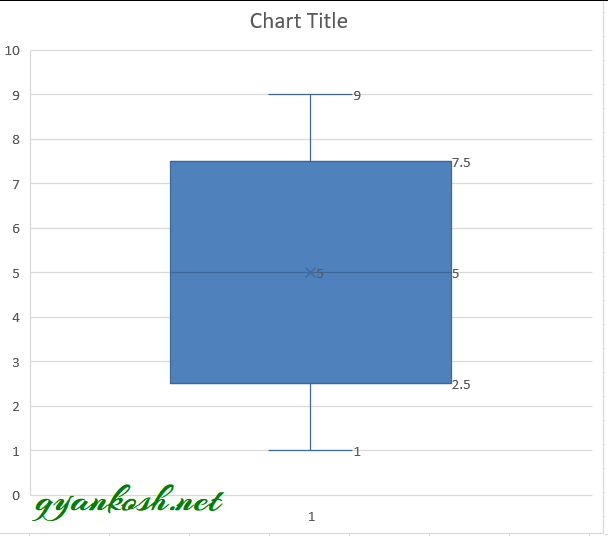PROVIDING ACCURATE, RELIABLE AND STRAIGHT TO THE POINT KNOWLEDGE ABOUT DIFFERENT IT TOOLS EXPLAINED WITH PRACTICAL EXAMPLES

# CREATE BOX AND WHISKER CHART IN EXCEL

## INTRODUCTION

CHARTS are the graphic representation of any data . As we know that EXCEL is a super analytical tool.

Analysis of data is the process of deriving the inferences by finding out the trends, averages etc. about different parameters.

Excel provides us with a variety of charts and graphs to make our life easier. Although any chart can be used for any type of data, even then there are certain types which are apt for a particular type of data.

This is a standard excerpt for the charts.

Now, in this article , we will discuss about the BOX AND WHISKER CHARTS which are specifically designed to present many statistical values at once which are very helpful in comparison of the data.

The BOX AND WHISKER has been given such name as it contains a BOX and the two lines are behaving like WHISKERS which gives some more information about the chart.

The upper end of the whisker gives the highest value in the series.

The lower end of the whisker gives the lowest value in the series.

A horizontal line shows the median of the data and a symbol X shows the average of the data.

The portion above the horizontal line is UPPER QUARTILE and lower of the line is LOWER QUARTILE.

So, we can see that this type of chart gave us FIVE DIFFERENT STATISTICAL INFORMATION at once.

## WHEN TO USE BOX AND WHISKER CHARTS

BOX AND WHISKER CHART provide us a few statistical information like maximum, minimum, median , upper quartile, lower quartile  of the data. We can use this type of chart if we need to compare this data for different sets of information or series.
It is really very easy to create and use this kind of chart .

## BUTTON LOCATION FOR SUNBURST CHARTS

The button for column chart is found under the INSERT TAB >CHARTS SECTION under the button INSERT STATISTICAL CHARTS >BOX AND WHISKER CHART. The location is shown in the picture below.

## KNOW THE STRUCTURE OF BOX AND WHISKER CHART

Before we start the example of the box and whisker chart, let us understand its structure.

### DIFFERENT PARTS OF A BOX AND WHISKER CHART IN EXCEL:

•  MAXIMUM- Highest value in the data set.
• MINIMUM- Lowest value in the data set.
• MEDIAN- median of the data
• MEAN- Average of the data
• 75TH AND 25TH PERCENTILE- Values at 25th percentile of the data and 75th percentile of the data which are shown as the box in the box and whisker chart.

## STEPS TO INSERT A BOX AND WHISKER CHART IN EXCEL

### EXAMPLE DETAILS

We can demonstrate the chart using an example.
We are taking the example of a CAR AGENCY which shows the sales details of three months.
The chart shows the data.
 VEHICLE SALE DATA TEAM APRIL MAY JUNE ABC 43 77 85 DEF 32 95 84 XYZ 12 90 62 DEF 54 76 86 ABC 66 65 86 XYZ 65 87 67 DEF 54 71 97 XYZ 34 58 94 ABC 23 61 89 ABC 45 79 52 ABC 65 98 96 XYZ 67 94 83 DEF 98 64 84 ABC 78 69 79 XYZ 67 73 57 DEF 56 78 90 ABC 45 92 72 DEF 34 85 52 XYZ 23 56 55 DEF 23 88 56 ABC 45 74 72

The procedure to insert a BOX AND WHISKER chart are as follows:

### STEPS TO INSERT A BOX AND WHISKER CHART IN EXCEL:

• The first requirement of any chart is data. So create a table containing the data.[We have already created in the form of table above]
• Refer to our data above, we have the sales numbers of three teams ABC, DEF and XYZ in a car agency.
• Select the complete table including the HEADER NAMES.
• Go to INSERT TAB> CHARTS> and click the BOX AND WHISKER CHART under  INSERT STATISTICAL CHARTS BUTTON as shown in the BUTTON LOCATION above and in the following picture for reference.
•• The chart will be created and shown to you as the following figure.

The complete process is shown in the animated picture below.

So , we have successfully created a BOX AND WHISKER CHART.

We can see that the data of all the teams for all the months has been plotted. The data is really easy to interpret and to compare. The highest and lowest values can be checked up easily.

The data can be compared easily too. The number is shown by the size of the box which can also be checked easily.

After applying styles and different layouts, here is our final chart.

The final chart is shown below.

## INTERPRETATION OF A BOX AND WHISKER CHART OR BOX PLOT

The BOX PLOT or BOX AND WHISKER CHART is easy to interpret.
The whiskers simply tell the maximum and minimum values.
The HORIZONTAL LINE represents the median of the data.

Let us take an example and solve it manually as well as with Excel.
Suppose we have a series as
1 2 3 4 5 6 7 8 9
We know that median of n number of series is nth number or nth+1 number.
We have 9 terms so median is the (9+1)/2 5th term which is 5.
Now we need to find out the 25th percentile.
PERCENTILE CAN BE FOUND WITH THE FOLLOWING FORMULA.
nth percentile =n/100 x (number of terms +1)
25th percentile=0.25×10=2.5
EXCEL would take it as exact 2.5 although the term number 2.5 is not possible so we can take it as 2 or 3. Let us take it as 2.
Similarly 75th percentile = 7.5 , We take it as 7.
Now we can see that 25 pc of the values are less than the value for lower quartile and 75 percent values are less than value shown in higher quartile.

The maximum and minimum are simple to identify which are 9 and 1 in our case.
Let us try this with Excel.## NOTE:CHANGING THE NAME OF THE CHART, CHANGING THE AXIS , CHANGING THE CHART STYLE ETC.

FOR ALL OTHER TASKS LIKE CHANGING THE NAME OF THE CHART, CHANGING THE AXIS , CHANGING THE CHART STYLE ETC. VISIT HERE [HOW TO CREATE A CHART IN EXCEL].

• ## WHAT IS EXCEL, HOW EXCEL WORKS, WRITE FORMULA IN EXCEL, EXAMPLE OF USING FORMULA IN EXCEL, ANIMATED EXAMLPE OF USING FORMULA IN EXCEL

LEARN

• EXCEL
• JAVASCRIPT
• MORE TO COME…

Encourage us by following at …

If you have any queries or suggestions or any problem which you would like us to solve for you, kindly mail at

gyankosh060309@gmail.com